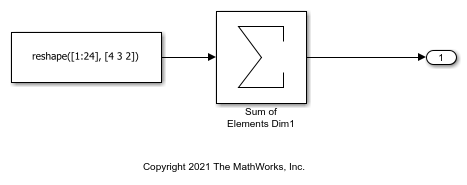# Row-Major Algorithms for Row-Major Array Layout

This example shows how to use row-major algorithms to generate efficient code. You can enable the Use algorithms optimized for row-major array layout configuration parameter to enable efficient row-major algorithms that are optimized for row-major array layout. The code that you generate by using row-major algorithms performs with better speed and efficient memory usage when operating on data with row-major array layout.

In this example, you operate on row-major data by first using the default column-major algorithms, and then using the row-major algorithms. This comparison helps in identifying the appropriate algorithm settings to achieve different requirements.

### Generate Code by Using the Default Column-Major Algorithms for Row-Major Array Layout

Open the example model `ex_rowmajor_algorithm`.

```model = 'ex_rowmajor_algorithm'; open_system(model); ```The model contains a Sum of Elements block and the input of the block is an array. By default, Simulink® configures a model with column-major algorithms and column-major array layout. In this example, you configure the array layout of this model as row-major. To specify the array layout, open the Embedded Coder app, and then open the Configuration Parameters dialog box. On the Code Generation > Interface pane, set the configuration parameter Array layout to `Row-Major` option. Alternatively, in the MATLAB® Command Window, enter:

```set_param(model,'ArrayLayout','Row-major'); ```

Change your current folder in MATLAB to a writable folder. Then generate code from the model by using the `slbuild` function or by pressing Ctrl+B.

```currentDir = pwd; [~,cgDir] = rtwdemodir(); % Generate code and capture the code generation information to Value Value=evalc('slbuild(''ex_rowmajor_algorithm'')'); ```

Inspect the generated `ex_rowmajor_algorithm_step` step function in the `ex_rowmajor_algorithm.c`.

```file = fullfile('ex_rowmajor_algorithm_ert_rtw','ex_rowmajor_algorithm.c'); rtwdemodbtype(file,'/* Model step function */','/* Model initialize function',1,1); ```
```/* Model step function */ void ex_rowmajor_algorithm_step(void) { int32_T i; int32_T i_0; int32_T tmp; /* Sum: '<Root>/Sum of Elements Dim1' incorporates: * Constant: '<Root>/Constant1' */ ex_rowmajor_algorithm_Y.Out2 = -0.0F; for (i = 0; i < 2; i++) { for (i_0 = 0; i_0 < 3; i_0++) { tmp = (i_0 << 1) + i; ex_rowmajor_algorithm_Y.Out2 = (((ex_rowmajor_algorithm_ConstP.Constant1_Value[tmp] + ex_rowmajor_algorithm_Y.Out2) + ex_rowmajor_algorithm_ConstP.Constant1_Value[tmp + 6]) + ex_rowmajor_algorithm_ConstP.Constant1_Value[tmp + 12]) + ex_rowmajor_algorithm_ConstP.Constant1_Value[tmp + 18]; } } /* End of Sum: '<Root>/Sum of Elements Dim1' */ } ```

When Array layout is set to `Row-major` and the Use algorithms optimized for row-major array layout configuration parameter is set to `off`, the code generator uses column-major algorithms. The algorithms traverse the data in column-major order though the data is in row-major order. This process requires some extra operations in the generated code, which makes the code less efficient. When the Use algorithms optimized for row-major array layout parameter is set to `off`, the code generator does not include other vectorization optimizations such as single-instance, multiple-data (SIMD) optimizations.

### Generate Code by Using the Row-Major Algorithms for Row-Major Array Layout

To enable the row-major algorithms, on the Math & Data Types pane, select the configuration parameter Use algorithms optimized for row-major array layout check box. This parameter enables the algorithms that are optimized for row-major array layout. Alternatively, in the MATLAB Command Window, enter:

```set_param(model,'UseRowMajorAlgorithm','on'); ```

After enabling the row-major algorithms, generate code.

```Value=evalc('slbuild(''ex_rowmajor_algorithm'')'); ```

Inspect the generated `ex_rowmajor_algorithm_step` step function in the `ex_rowmajor_algorithm.c`.

```file = fullfile('ex_rowmajor_algorithm_ert_rtw','ex_rowmajor_algorithm.c'); rtwdemodbtype(file,'/* Model step function */','/* Model initialize function',1,1); ```
```/* Model step function */ void ex_rowmajor_algorithm_step(void) { int32_T i; /* Sum: '<Root>/Sum of Elements Dim1' incorporates: * Constant: '<Root>/Constant1' */ ex_rowmajor_algorithm_Y.Out2 = -0.0F; for (i = 0; i < 24; i++) { ex_rowmajor_algorithm_Y.Out2 += ex_rowmajor_algorithm_ConstP.Constant1_Value[i]; } /* End of Sum: '<Root>/Sum of Elements Dim1' */ } ```

When the Array layout is set to `Row-major` and the Use algorithms optimized for row-major array layout configuration parameter is set to `on`, the code generator generates efficient code. The code generator uses row-major algorithms that traverse the data in row-major order, which reduces the number of operations in the generated code. However, you might notice a minor numeric difference between the outputs of column-major and row-major algorithms due to the operation order.